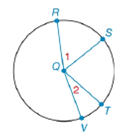Chapter 6.4, Problem 18E### Elementary Geometry for College St...

6th Edition
Daniel C. Alexander + 1 other
ISBN: 9781285195698

#### Solutions

Chapter
Section### Elementary Geometry for College St...

6th Edition
Daniel C. Alexander + 1 other
ISBN: 9781285195698
Textbook Problem
1 views

# a) If m R S ⌢ > m T V ⌢ , write an inequality that compares m ∠ 1 with m ∠ 2 .b) If m ∠ 1 > m ∠ 2 , write and inequality that compares m R S ⌢ with m T V ⌢ .To determine

(a)

To write:

An inequality that compares m1 with m2.

Explanation

Given:

mRS>mTV and the figure given below

Theorem:

In a circle (or in congruent circles) containing two unequal arcs, the larger arc corresponds to the larger central angle.

Calculation:

From the theorem,

Since the arcs are unequal i.e. mRS>mTV and from the given figure,

To determine

(b)

To write:

An inequality that compares mRS with mTV.

### Still sussing out bartleby?

Check out a sample textbook solution.

See a sample solution

#### The Solution to Your Study Problems

Bartleby provides explanations to thousands of textbook problems written by our experts, many with advanced degrees!

Get Started

#### Find more solutions based on key concepts